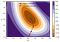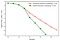# Newton’s Method vs. Gradient descent

## Newton = Gradient + Hessian

See https://medium.com/@ranjeettate/optimal-learning-rate-from-the-hessian-examples-e89f8d1af977 for some background. See https://en.wikipedia.org/wiki/Newton%27s_method_in_optimization for a complete derivation of Newton’s Method and how it uses the second derivative (the Hessian) to improve Gradient descent.

Post in progress, but meanwhile, here are the pictures:Hybrid and gradient descent paths on the contour plotFunction values approaching the minimum

Key part of the code for hybrid descent, note that it includes Gradient descent as well as Newton’s Method (or what I was calling Hessian descent), code for which I haven’t included separately.

`def hybrid_descent(foo, initial_point, iterations = 5, damping = 0.25):    point = initial_point    dim = len(initial_point)    # gradient of foo    dfoo = nd.Gradient(foo)    iterL = []    for iter in range(iterations):        iterD = {}        function_value = foo(point)# gradient of foo at the current iteration point        gradient = tf.reshape(dfoo(point), shape = [dim,1])        # practical gradient change in the variables, note the default damping = 0.25        delta_grad = - gradient * damping# Hessian (matrix of mixed partial derivatives) of foo        hessian = tf.constant(nd.Hessian(f)(point))        # inverse of the Hessian        inv_hessian = tf.matrix_inverse(hessian, adjoint=False)        # optimal vector change in the variables, note the '-'         optimal_delta = -tf.matmul(inv_hessian, gradient)        # practical Hessian vector change in the variables, note the Hessian damping = 2 * damping for gradient        delta_hess = optimal_delta * damping * 2        # Hessian "norm" of (negative) gradient = optimal_delta * ( - gradient), ?> 0        # = Hessian **(-1) * gradient * gradient        hess_grad_sq = - tf.matmul(tf.reshape(optimal_delta, shape = [1, dim]), gradient)# reshape        gradient = tf.reshape(gradient, shape = [dim,])        delta_grad = tf.reshape(delta_grad, shape = [dim,])        delta_hess = tf.reshape(delta_hess, shape = [dim,])        hess_grad_sq = tf.reshape(hess_grad_sq, shape = [1,])with tf.Session() as sess:            # sess.run(tf.global_variables_initializer())            gradient = sess.run(gradient)            hess_grad_sq = sess.run(hess_grad_sq)            if hess_grad_sq > 0:                 delta = sess.run(delta_hess)                method = 'Hessian'            else:                delta = sess.run(delta_grad)                method = 'Gradient'            iterD = {"iteration": iter,                     "function_value": function_value,                     "point": map(lambda x: round(x, 3), point),                     "gradient": map(lambda x: round(x, 3), gradient),                     "method": method,                     "delta": map(lambda x: round(x, 3), delta),                     "Hessian_Gradient_Sq": hess_grad_sq}        iterL.append(iterD)        point = map(lambda i: point[i] + delta[i], range(dim))    hybrid_descentDF = pd.DataFrame(iterL)    return hybrid_descentDF`

## More from Ranjeet Tate

I stop to miau to cats.